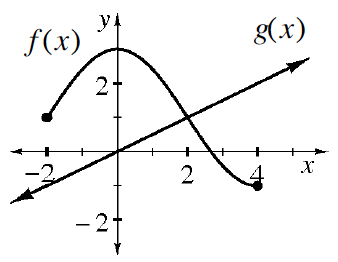### Home > GB8I > Chapter 11 Unit 12 > Lesson INT1: 11.2.5 > Problem11-109

11-109.

Examine the graph of functions $f(x)$ and $g(x)$ at right. Use the graph to approximate the values below (if possible).1. $f\left(0\right)$

When $x$ is $0$ in the relation $f\left(x\right)$, what is $y$?

$f\left(0\right) = 3$

1. $g(4)$

When $x$ is $4$ in the relation $g\left(x\right)$, what is $y$?

$g\left(4\right) = 2$

1. $f(2)$

See the hint for part (a).

1. $g(0)$

See the hint for part (b).

1. $g(2)$

See the hint for part (b).

1. $f(5)$

Is there a $y$-value for $f\left(x\right)$ when $x$ is $5$?

1. $x\text{ such that}f(x)=g(x)$

For what $x$ value are the two functions equal?